Blob Farm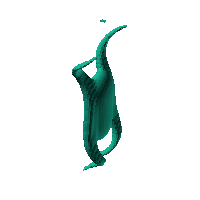# blob12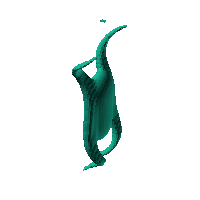The blob in all its glory: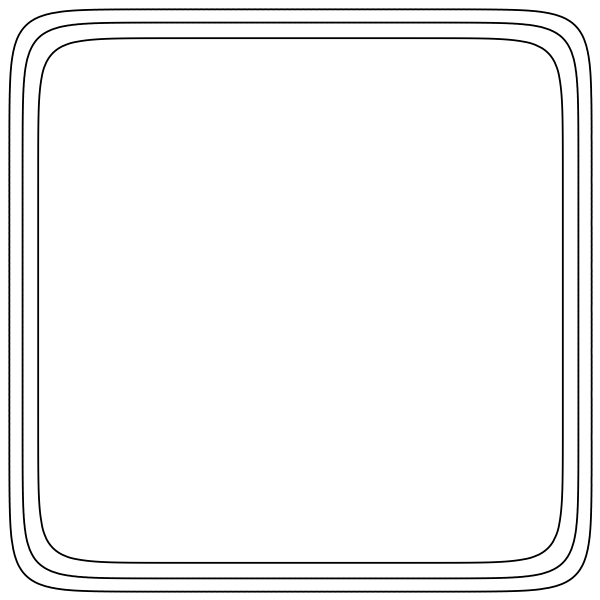Inspired by a shark egg case, trying to head toward a pencil case.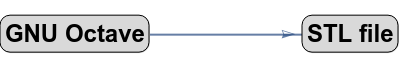Click on the snapshot to download the blob's stl file.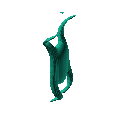Octave Code:
```1; # Prevent Octave from thinking that this is a function
# though one is defined here

function w = f(x2,y2,z2,c,r,e)
x  = (x2-c(1))/r(1);
y  = (y2-c(2))/r(2);
z  = (z2-c(3))/r(3);

xp = x+sin((z*0.5).^2).*z*0.2;
yp = y+cos((z*0.5).^2).*z*0.2;
yp2 =  -y+cos((z*0.5).^2).*z*0.2;
zp = z;

# function at origin must be <0, and >0 far enough away.  w=0 defines the surface
w = (1.0)./(((((1/2)*z-0)).^4+(1.66*x).^2+(1*y).^2)+0.01);
w = w + (1.0)./((((1/4)*zp).^2+(5*xp).^4+(3*(yp-1.2)).^4)+0.01);
w = w + (1.0)./((((1/4)*zp).^2+(5*xp).^4+(3*(yp2-1.2)).^4)+0.01);
w = w- 1.0;
endfunction;

```
GNU Octave